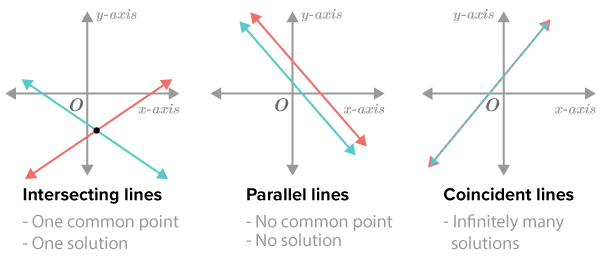Enrol now for our new online tutoring program. Learn from the best tutors. Get amazing results. Learn more.

June 26, 2022, 12:40:13 pm

###AuthorTopic: maths help  (Read 1066 times) Tweet Share

0 Members and 1 Guest are viewing this topic.

#### happyy

•• Posts: 5
• Respect: 0##### maths help
« on: February 21, 2022, 09:51:33 pm »
+1
hey AN, im trying to find the value of k for which the simultaneous linear equations below have a. infinitely many solutions, b. unique solution and c. no solutions
I am getting confused on how im supposed to know which values us correct for each solution. can someone pls provide me working out and explanations?
thankyou

https://imgur.com/af6XPNE

#### 1729

• MOTM: July 20
• Trendsetter
•• Posts: 178
• Respect: +151##### Re: maths help
« Reply #1 on: February 22, 2022, 07:08:25 pm »
+5
hey AN, im trying to find the value of k for which the simultaneous linear equations below have a. infinitely many solutions, b. unique solution and c. no solutions
I am getting confused on how im supposed to know which values us correct for each solution. can someone pls provide me working out and explanations?
thankyou
Hi happyy!
For easier reference I'm going to repost the question

Question
Consider the simultaneous linear equations where $k$ is a real constant.
$kx-4y=k+3$
$4x+\left(k+10\right)y=-2$

Find the values of $k$ for which the simultaneous equations have

Note: To help better explain this, let's rearrange the two lines in $y=mx+c$ form. This will respectively yield to

$y=-\frac{4}{k+10}x-\frac{2}{k+10}$
$y=\frac{k}{4}x-\frac{k+3}{4}$

$\mathbf{a.}\quad$ Infinitely many solutions

Solution
The condition for there to be infinitely many solutions means that the two lines are identical.
For the lines to be identical, there are two condtions - the gradients and $y$ intercepts must also be identical, that is
$\frac{k}{4}=-\frac{4}{k+10}$ which gets solutions $k=-2,\:k=-8$
We must also solve for $y$ intercepts to determine which solutions satisfy both conditions
$\frac{k+3}{4}=\frac{2}{k+10}$ which gets solutions $k=-2,\:k=-11$
Clearly, $k=-2$ satisfies both conditions, therefore there will be infinite number of solutions when $k=-2$

$\mathbf{b.}\quad$ One unique solution

Solution
There will be exactly one unique solution when the gradients are different.
Above we figured out the gradients will be equal when $k=-2,\:k=-8$, therefore they will be different everywhere else.
Therefore, there will be one unique solution when $k\ne -2,\:k\ne -8$ or $k\in \mathbb{R}\:\backslash \left\{-2,\:-8\right\}$
$\mathbf{c.}\quad$ No solutions

Solution
There will be no solutions when these two lines are parallel.
We figured out the gradients are the same when $k=-2,\:k=-8$, however, when $k=-2$ there are infinitely many solutions (they not only have the same gradient but they also have the same equation)
So therefore, there will be no solution when $k=-8$.

The diagram below might also help understanding this explanation